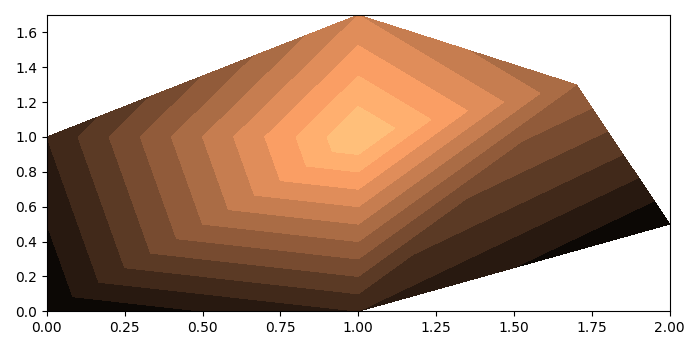# How to plot 2d FEM results using matplotlib?

The Finite Element Method (FEM) is used in a variety of tasks such as modeling of different material types, testing complex geometries, visualizing the local effects acting on a small area of a design. It basically breaks a large spatial domain into simple parts called "finite elements". The simple equations that model these finite elements are then collected into a larger system of equations to model the entire domain.

To plot 2d FEM results using matplotlib, we can take the following steps −

• Set the figure size and adjust the padding between and around the subplots.
• Create nodes, elements and node values data points using numpy.
• Transpose the nodes' data points.
• Create a 3D filled contour plot, using tricontourf().
• To display the figure, use show() method.

## Example

import numpy as np
import matplotlib.pyplot as plt

plt.rcParams["figure.figsize"] = [7.00, 3.50]
plt.rcParams["figure.autolayout"] = True

nodes = np.array([
[0.0, 0.0],
[1.0, 0.0],
[2.0, 0.5],
[0.0, 1.0],
[1.0, 1.0],
[1.7, 1.3],
[1.0, 1.7]])

elements = np.array([
[1, 2, 5],
[5, 4, 1],
[2, 3, 6],
[6, 5, 2],
[4, 5, 7],
[5, 6, 7]])

values = [1, 2, 1, 2, 7, 4, 5]

x, y = nodes.T

plt.tricontourf(x, y, elements - 1, values, 12, cmap='copper')

plt.show()

## Output

It will produce the following output